xCylinder (geometry)Overview

A cylinder is one of the most basic curvilinear geometric shapes, the surface
Surface
In mathematics, specifically in topology, a surface is a two-dimensional topological manifold. The most familiar examples are those that arise as the boundaries of solid objects in ordinary three-dimensional Euclidean space R3 — for example, the surface of a ball...

formed by the points at a fixed distance from a given line segment
Line segment
In geometry, a line segment is a part of a line that is bounded by two end points, and contains every point on the line between its end points. Examples of line segments include the sides of a triangle or square. More generally, when the end points are both vertices of a polygon, the line segment...

, the axis of the cylinder. The solid enclosed by this surface and by two planes perpendicular to the axis is also called a cylinder. The surface area
Surface area
Surface area is the measure of how much exposed area a solid object has, expressed in square units. Mathematical description of the surface area is considerably more involved than the definition of arc length of a curve. For polyhedra the surface area is the sum of the areas of its faces...

and the volume
Volume
Volume is the quantity of three-dimensional space enclosed by some closed boundary, for example, the space that a substance or shape occupies or contains....

of a cylinder have been known since deep antiquity.

In differential geometry, a cylinder is defined more broadly as any ruled surface
Ruled surface
In geometry, a surface S is ruled if through every point of S there is a straight line that lies on S. The most familiar examples are the plane and the curved surface of a cylinder or cone...

spanned by a one-parameter family of parallel lines.Unanswered QuestionsIs it possible to project a square (or rectangular) silhouette of a cylinder?EncyclopediaA cylinder is one of the most basic curvilinear geometric shapes, the surface
Surface
In mathematics, specifically in topology, a surface is a two-dimensional topological manifold. The most familiar examples are those that arise as the boundaries of solid objects in ordinary three-dimensional Euclidean space R3 — for example, the surface of a ball...

formed by the points at a fixed distance from a given line segment
Line segment
In geometry, a line segment is a part of a line that is bounded by two end points, and contains every point on the line between its end points. Examples of line segments include the sides of a triangle or square. More generally, when the end points are both vertices of a polygon, the line segment...

, the axis of the cylinder. The solid enclosed by this surface and by two planes perpendicular to the axis is also called a cylinder. The surface area
Surface area
Surface area is the measure of how much exposed area a solid object has, expressed in square units. Mathematical description of the surface area is considerably more involved than the definition of arc length of a curve. For polyhedra the surface area is the sum of the areas of its faces...

and the volume
Volume
Volume is the quantity of three-dimensional space enclosed by some closed boundary, for example, the space that a substance or shape occupies or contains....

of a cylinder have been known since deep antiquity.

In differential geometry, a cylinder is defined more broadly as any ruled surface
Ruled surface
In geometry, a surface S is ruled if through every point of S there is a straight line that lies on S. The most familiar examples are the plane and the curved surface of a cylinder or cone...

spanned by a one-parameter family of parallel lines. A cylinder whose cross section
Cross section (geometry)
In geometry, a cross-section is the intersection of a figure in 2-dimensional space with a line, or of a body in 3-dimensional space with a plane, etc...

is an ellipse
Ellipse
In geometry, an ellipse is a plane curve that results from the intersection of a cone by a plane in a way that produces a closed curve. Circles are special cases of ellipses, obtained when the cutting plane is orthogonal to the cone's axis...

, parabola
Parabola
In mathematics, the parabola is a conic section, the intersection of a right circular conical surface and a plane parallel to a generating straight line of that surface...

, or hyperbola
Hyperbola
In mathematics a hyperbola is a curve, specifically a smooth curve that lies in a plane, which can be defined either by its geometric properties or by the kinds of equations for which it is the solution set. A hyperbola has two pieces, called connected components or branches, which are mirror...

is called an elliptic cylinder, parabolic cylinder, or hyperbolic cylinder respectively.

## Common use

In common use a cylinder is taken to mean a finite section of a right circular cylinder, i.e., the cylinder with the generating lines perpendicular to the bases, with its ends closed to form two circular surfaces, as in the figure (right). If the cylinder has a radius
Radius
In classical geometry, a radius of a circle or sphere is any line segment from its center to its perimeter. By extension, the radius of a circle or sphere is the length of any such segment, which is half the diameter. If the object does not have an obvious center, the term may refer to its...

and length (height) , then its volume
Volume
Volume is the quantity of three-dimensional space enclosed by some closed boundary, for example, the space that a substance or shape occupies or contains....

is given by

and its surface area
Surface area
Surface area is the measure of how much exposed area a solid object has, expressed in square units. Mathematical description of the surface area is considerably more involved than the definition of arc length of a curve. For polyhedra the surface area is the sum of the areas of its faces...

is:
• the area of the top
• the area of the bottom
• the area of the side .

Therefore without the top or bottom (lateral area), the surface area is:
.

With the top and bottom, the surface area is:
.

For a given volume, the cylinder with the smallest surface area has . For a given surface area, the cylinder with the largest volume has , i.e. the cylinder fits in a cube (height = diameter).

## Volume

Having a right circular cylinder with a height units and a base of radius units with the coordinate axes chosen so that the origin is at the center of one base and the height is measured along the positive x-axis. A plane section at a distance of units from the origin has an area of square units where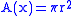or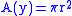An element of volume, is a right cylinder of base area square units and a thickness of units. Thus if cubic units is the volume of the right circular cylinder, by Riemann sums,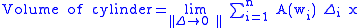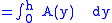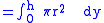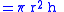Using cylindrical coordinates, the volume can be calculated by integration over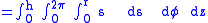## Cylindric section

Cylindric sections are the intersections of cylinders with planes. For a right circular cylinder, there are four possibilities. A plane tangent to the cylinder, meets the cylinder in a single straight line. Moved while parallel to itself, the plane either does not intersect the cylinder or intersects it in two parallel lines. All other planes intersect the cylinder in an ellipse or, when they are perpendicular to the axis of the cylinder, in a circle.

## Other types of cylinders

An elliptic cylinder, or cylindroid
Cylindroid
A cylindroid is a cylinder that has an elliptical cross-section. See also Plücker’s conoid....

, is a quadric surface, with the following equation in Cartesian coordinates: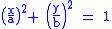This equation is for an elliptic cylinder, a generalization of the ordinary, circular cylinder . Even more general is the generalized cylinder: the cross-section
Cross section (geometry)
In geometry, a cross-section is the intersection of a figure in 2-dimensional space with a line, or of a body in 3-dimensional space with a plane, etc...

can be any curve.

The cylinder is a degenerate quadric
Quadric
In mathematics, a quadric, or quadric surface, is any D-dimensional hypersurface in -dimensional space defined as the locus of zeros of a quadratic polynomial...

because at least one of the coordinates (in this case ) does not appear in the equation.

An oblique cylinder has the top and bottom surfaces displaced from one another.

There are other more unusual types of cylinders. These are the imaginary elliptic cylinders: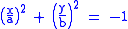the hyperbolic cylinder: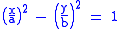and the parabolic cylinder: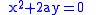## About an arbitrary axis

To describe the cylindrical surface surrounding an arbitrary axis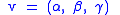We make use of spherical coordinates: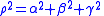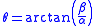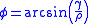These variables can be used to define A and B, the orthogonal vectors that form the basis for the cylinder: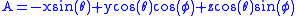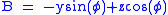With these defined, we may use the familiar formula for a cylinder:where R is the radius of the cylinder.
These results are usually derived using rotation matrices.

## Projective geometry

In projective geometry
Projective geometry
In mathematics, projective geometry is the study of geometric properties that are invariant under projective transformations. This means that, compared to elementary geometry, projective geometry has a different setting, projective space, and a selective set of basic geometric concepts...

, a cylinder is simply a cone
Cone (geometry)
A cone is an n-dimensional geometric shape that tapers smoothly from a base to a point called the apex or vertex. Formally, it is the solid figure formed by the locus of all straight line segments that join the apex to the base...

whose apex
Apex (geometry)
In geometry, an apex is the vertex which is in some sense the highest of the figure to which it belongs.*In an isosceles triangle, the apex is the vertex where the two sides of equal length meet, opposite the unequal third side....

is at infinity.

This is useful in the definition of degenerate conic
Degenerate conic
In mathematics, a degenerate conic is a conic that fails to be an irreducible curve...

s, which require considering the cylindrical conics.

## See also

• Steinmetz solid
Steinmetz solid
In geometry, the Steinmetz solid is the solid body generated by the intersection of two or three cylinders of equal radius at right angles. It is named after Charles Proteus Steinmetz, though these solids were known long before Steinmetz studied them....

, the intersection of two or three perpendicular cylinders
• Prism (geometry)
Prism (geometry)
In geometry, a prism is a polyhedron with an n-sided polygonal base, a translated copy , and n other faces joining corresponding sides of the two bases. All cross-sections parallel to the base faces are the same. Prisms are named for their base, so a prism with a pentagonal base is called a...

## External links

The source of this article is wikipedia, the free encyclopedia.  The text of this article is licensed under the GFDL.